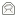## Overview

### Key Generation

1. Generate two large prime numbers, p and q
2. Let n = pq
3. Let m = (p-1)(q-1)
4. Choose a small number e, coprime to m
5. Find d, such that de % m = 1

Publish e and n as the public key.
Keep d and n as the secret key.

C = Pe % n

### Decryption

P = Cd % n
x % y means the remainder of x divided by y

The reasons why this algorithm works are discussed in the mathematics section. Its security comes from the computational difficulty of factoring large numbers. To be secure, very large numbers must be used for p and q - 100 decimal digits at the very least.

I'll now go through a simple worked example.

## Key Generation

### 1) Generate two large prime numbers, p and q

To make the example easy to follow I am going to use small numbers, but this is not secure. To find random primes, we start at a random number and go up ascending odd numbers until we find a prime. Lets have:

p = 7
q = 19

n = 7 * 19
= 133

### 3) Let m = (p - 1)(q - 1)

m = (7 - 1)(19 - 1)
= 6 * 18
= 108

### 4) Choose a small number, e coprime to m

e coprime to m, means that the largest number that can exactly divide both e and m (their greatest common divisor, or GCD) is 1. Euclid's algorithm is used to find the GCD of two numbers, but the details are omitted here.

e = 2 => GCD(e, 108) = 2 (no)
e = 3 => GCD(e, 108) = 3 (no)
e = 4 => GCD(e, 108) = 4 (no)
e = 5 => GCD(e, 108) = 1 (yes!)

### 5) Find d, such that de % m = 1

This is equivalent to finding d which satisfies `de = 1 + nm` where n is any integer. We can rewrite this as `d = (1 + nm) / e`. Now we work through values of n until an integer solution for e is found:

n = 0 => d = 1 / 5 (no)
n = 1 => d = 109 / 5 (no)
n = 2 => d = 217 / 5 (no)
n = 3 => d = 325 / 5
= 65 (yes!)

To do this with big numbers, a more sophisticated algorithm called extended Euclid must be used.

n = 133
e = 5

n = 133
d = 65

## Communication

### Encryption

The message must be a number less than the smaller of p and q. However, at this point we don't know p or q, so in practice a lower bound on p and q must be published. This can be somewhat below their true value and so isn't a major security concern. For this example, lets use the message "6".

C = Pe % n
= 65 % 133
= 7776 % 133
= 62

### Decryption

This works very much like encryption, but involves a larger exponentiation, which is broken down into several steps.

P = Cd % n
= 6265 % 133
= 62 * 6264 % 133
= 62 * (622)32 % 133
= 62 * 384432 % 133
= 62 * (3844 % 133)32 % 133
= 62 * 12032 % 133

We now repeat the sequence of operations that reduced 6265 to 12032 to reduce the exponent down to 1.

= 62 * 3616 % 133
= 62 * 998 % 133
= 62 * 924 % 133
= 62 * 852 % 133
= 62 * 43 % 133
= 2666 % 133
= 6

And that matches the plaintext we put in at the beginning, so the algorithm worked!

© 1998 - 2012Paul Johnston, distributed under the BSD License   Updated:16 Jul 2009